The OEIS Foundation is supported by donations from users of the OEIS and by a grant from the Simons Foundation.Hints (Greetings from The On-Line Encyclopedia of Integer Sequences!)

## (Page 12)## Fractions, Arrays, Real Numbers, etc.

The OEIS contains much more than just sequences of whole numbers. There are also sequences of fractions or rational numbers, triangles or other two-dimensional arrays of numbers, decimal expansions and continued fraction expansions of important constants, among other things.## Rational Numbers or Fractions

• Rational numbers (or fractions) are given as a pair of sequences, listing the numerators and denominators separately.
• This is indicated by the keyword "frac", and the two sequences are cross-referenced to each other.
• The Bernoulli numbers are a good example. This is probably the most important sequence of fractions in number theory. It begins: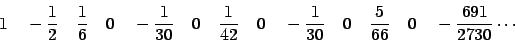• The numerators and denominators are listed separately as sequences A027641 and A027642 as shown here.
• Note that there is a separate file http://oeis.org/wiki/Index_to_fractions_in_OEIS that gives the A-numbers of the numerator and denominator sequences. for a number of important sequences of rational numbers.First the numerator sequence:

 A027641 Numerator of Bernoulli number B_n. 73
 1, -1, 1, 0, -1, 0, 1, 0, -1, 0, 5, 0, -691, 0, 7, 0, -3617, 0, 43867, 0, -174611, 0, 854513, 0, -236364091, 0, 8553103, 0, -23749461029, 0, 8615841276005, 0, -7709321041217, 0, 2577687858367, 0, -26315271553053477373, 0, 2929993913841559, 0, -261082718496449122051 (list; graph; refs; listen; history; edit; internal format)Then the denominators

 A027642 Denominator of Bernoulli number B_n. +026
 1, 2, 6, 1, 30, 1, 42, 1, 30, 1, 66, 1, 2730, 1, 6, 1, 510, 1, 798, 1, 330, 1, 138, 1, 2730, 1, 6, 1, 870, 1, 14322, 1, 510, 1, 6, 1, 1919190, 1, 6, 1, 13530, 1, 1806, 1, 690, 1, 282, 1, 46410, 1, 66, 1, 1590, 1, 798, 1, 870, 1, 354, 1, 56786730, 1 (list)
 OFFSET 0,2 REFERENCES M. Abramowitz and I. A. Stegun, eds., Handbook of Mathematical Functions, National Bureau of Standards Applied Math. Series 55, 1964 (and various reprintings), p. 810. .....................................................
 page 1## Two-dimensional Arrays of Numbers

• Many familiar numbers are not part of of a sequence but rather belong to a two-dimensional array. These are entered into the database by reading them by rows (or antidiagonals in the case of square arrays) to convert them to one-dimensional sequences.
• Perhaps the most familiar array is Pascal's Triangle of binomial coefficients C(n,k) = n! / (k! (n-k)!) :
 1 1 1 1 2 1 1 3 3 1 1 4 6 4 1 1 5 10 10 5 1 1 6 15 20 15 6 1 1 7 21 35 35 21 7 1 ... ... ... ... ... ... ... ...

• Reading this by rows produces the following sequence.A007318 Pascal's triangle read by rows: C(n,k) = binomial(n,k) = n!/(k!*(n-k)!), 0<=k<=n. (Formerly M0082) 1175
 1, 1, 1, 1, 2, 1, 1, 3, 3, 1, 1, 4, 6, 4, 1, 1, 5, 10, 10, 5, 1, 1, 6, 15, 20, 15, 6, 1, 1, 7, 21, 35, 35, 21, 7, 1, 1, 8, 28, 56, 70, 56, 28, 8, 1, 1, 9, 36, 84, 126, 126, 84, 36, 9, 1, 1, 10, 45, 120, 210, 252, 210, 120, 45, 10, 1, 1, 11, 55, 165, 330, 462, 462, 330, 165, 55, 11, 1 (list; table; graph; refs; listen; history; edit; internal format)• The keyword "tabl" indicates a sequence formed from a triangular or square array of numbers.
Clicking on this keyword produces a two-dimensional display of the sequence:A007318 formatted as a triangular array:

 1 1 1 1 2 1 1 3 3 1 1 4 6 4 1 1 5 10 10 5 1 1 6 15 20 15 6 1 1 7 21 35 35 21 7 1 1 8 28 56 70 56 28 8 1 1 9 36 84 126 126 84 36 9 1 1 10 45 120 210 252 210 120 45 10 1 1 11 55 165 330 462 462 330 165 55 11 1## Important Constants

• The database also contains many important constants (e,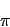, the speed of light, etc.).
• These are usually represented in two ways:
• as a sequence of decimal digits, and
• as a continued fraction expansion
The former is the more familiar representation, while the latter is less dependent on our base 10 decimal notation.
• For example, here are the two sequences representing the classical constant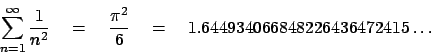First the decimal expansion:

 A013661 Decimal expansion of zeta(2) = Pi^2/6. 34
 1, 6, 4, 4, 9, 3, 4, 0, 6, 6, 8, 4, 8, 2, 2, 6, 4, 3, 6, 4, 7, 2, 4, 1, 5, 1, 6, 6, 6, 4, 6, 0, 2, 5, 1, 8, 9, 2, 1, 8, 9, 4, 9, 9, 0, 1, 2, 0, 6, 7, 9, 8, 4, 3, 7, 7, 3, 5, 5, 5, 8, 2, 2, 9, 3, 7, 0, 0, 0, 7, 4, 7, 0, 4, 0, 3, 2, 0, 0, 8, 7, 3, 8, 3, 3, 6, 2, 8, 9, 0, 0, 6, 1, 9, 7, 5, 8, 7, 0 (list; constant; graph; refs; listen; history; edit; internal format)
 OFFSET 1,2 COMMENTS Sum_{m = 1..inf } 1/m^2. "In 1736 he [Leonard Euler, 1707-1783] discovered the limit to the infinite series, Sum 1/n^2. He did it by doing some rather ingenious mathematics using trigonometric functions that proved the series summed to exactly Pi^2/6. How can this be? ... This demonstrates one of the most startling characteristics of mathematics - the interconnectedness of, seemingly, unrelated ideas." - Clawson Also dilogarithm(1). - Rick L. Shepherd (rshepherd2(AT)hotmail.com), Jul 21 2004 Also Integral_{x=0..inf} x/(exp(x)-1). For the partial sums see the fractional sequence A007406/A007407. REFERENCES M. Abramowitz and I. A. Stegun, eds., Handbook of Mathematical Functions, National Bureau of Standards Applied Math. Series 55, 1964 (and various reprintings), p. 811. R. Calinger, "Leonard Euler: The First St. Petersburg Years (1727-1741)," Historia Mathematica, Vol. 23, 1996, pp. 121-166. Calvin C. Clawson, Mathematical Mysteries, The Beauty and Magic of Numbers, Perseus Books, 1996, p. 97. W. Dunham, Euler: The Master of Us All, The Mathematical Association of America, Washington, D.C., 1999, p. xxii. G. F. Simmons, Calculus Gems, Section B.15,B.24 pp. 270-1,323-5 McGraw Hill 1992. A. Weil, Number theory: an approach through history; from Hammurapi to Legendre, Birkhaeuser, Boston, 1984; see p. 261. David Wells, "The Penguin Dictionary of Curious and Interesting Numbers," Revised Edition, Penguin Books, London, England, 1997, page 23. LINKS Harry J. Smith, Table of n, a(n) for n=1,...,20000 M. Abramowitz and I. A. Stegun, eds., Handbook of Mathematical Functions, National Bureau of Standards, Applied Math. Series 55, Tenth Printing, 1972 [alternative scanned copy]. D. H. Bailey, J. M. Borwein and D. M. Bradley, Experimental determination of Ap'ery-like identities for zeta(4n+2) R. W. Clickery, Probability of two numbers being coprime [Broken link] L. Euler, On the sums of series of reciprocals L. Euler, De summis serierum reciprocarum, E41. Math. Reference Project, The Zeta Function, Zeta(2) Math. Reference Project, The Zeta Function, Odds That Two Numbers Are Coprime" J. Perry, Prime Product Paradox S. Plouffe, Plouffe's Inverter, Zeta(2) or Pi**2/6 to 100000 digits S. Plouffe, Zeta(2) or Pi**2/6 to 10000 places A. L. Robledo, PlanetMath.org, value of the Riemann zeta function at s=2 E. Sandifer, How Euler Did It, Estimating the Basel Problem E. Sandifer, How Euler Did It, Basel Problem with Integrals C. Tooth, Pi squared over six Eric Weisstein's World of Mathematics, Link to a section of The World of Mathematics Eric Weisstein's World of Mathematics, Dilogarithm MathWorld page FORMULA Limit(n-->+oo) of (1/n)*(sum(k=1, n, frac((n/k)^(1/2)))) = zeta(2) and in general have limit(n-->+oo) of (1/n)*(sum(k=1, n, frac((n/k)^(1/m)))) = zeta(m), m >= 2. - Yalcin Aktar (aktaryalcin(AT)msn.com), Jul 14 2005 EXAMPLE 1.6449340668482264364724151666460251892189499012067984377355582293700074704032... MATHEMATICA RealDigits[N[Pi^2/6, 100]][] PROG (PARI) \p 200; Pi^2/6 (PARI) \p 200 dilog(1) \p 200 zeta(2) (PARI) a(n)=if(n<1, 0, default(realprecision, n+2); floor(Pi^2/6*10^(n-1))%10) (PARI) { default(realprecision, 20080); x=Pi^2/6; for (n=1, 20000, d=floor(x); x=(x-d)*10; write("b013661.txt", n, " ", d)); } [From Harry J. Smith (hjsmithh(AT)sbcglobal.net), Apr 29 2009] CROSSREFS Cf. A013679, A013631, A013680, 1/A059956. Sequence in context: A195359 A021612 A110756 * A019174 A019166 A058158 Adjacent sequences:  A013658 A013659 A013660 * A013662 A013663 A013664 KEYWORD cons,nonn,nice,changed AUTHOR N. J. A. Sloane (njas(AT)research.att.com). EXTENSIONS Fixed my PARI program, had -n Harry J. Smith (hjsmithh(AT)sbcglobal.net), May 19 2009Then the continued fraction expansion:

 A013679 Continued fraction for zeta(2) = Pi^2/6. 4
 1, 1, 1, 1, 4, 2, 4, 7, 1, 4, 2, 3, 4, 10, 1, 2, 1, 1, 1, 15, 1, 3, 6, 1, 1, 2, 1, 1, 1, 2, 2, 3, 1, 3, 1, 1, 5, 1, 2, 2, 1, 1, 6, 27, 20, 3, 97, 105, 1, 1, 1, 1, 1, 45, 2, 8, 19, 1, 4, 1, 1, 3, 1, 2, 1, 1, 1, 5, 1, 1, 2, 3, 6, 1, 1, 1, 2, 1, 5, 1, 1, 2, 9, 5, 3, 2, 1, 1, 1 (list; graph; refs; listen; history; edit; internal format)
 OFFSET 1,5 REFERENCES M. Abramowitz and I. A. Stegun, eds., Handbook of Mathematical Functions, National Bureau of Standards Applied Math. Series 55, 1964 (and various reprintings), p. 811. David Wells, "The Penguin Dictionary of Curious and Interesting Numbers," Revised Edition, Penguin Books, London, England, 1997, page 23. LINKS T. D. Noe, Table of n, a(n) for n = 1..10000 M. Abramowitz and I. A. Stegun, eds., Handbook of Mathematical Functions, National Bureau of Standards, Applied Math. Series 55, Tenth Printing, 1972 [alternative scanned copy]. G. Xiao, Contfrac EXAMPLE 1.6449340668482264364724151666460251892189... 1.644934066848226436472415166... = 1 + 1/(1 + 1/(1 + 1/(1 + 1/(4 + ...)))) [From Harry J. Smith (hjsmithh(AT)sbcglobal.net), Apr 29 2009] MATHEMATICA ContinuedFraction[ Pi^2/6, 100] PROG (PARI) { allocatemem(932245000); default(realprecision, 21000); x=contfrac(Pi^2/6); for (n=1, 20000, write("b013679.txt", n, " ", x[n])); } [From Harry J. Smith (hjsmithh(AT)sbcglobal.net), Apr 29 2009] CROSSREFS Cf. A013661. Sequence in context: A007005 A066978 A114566 * A096428 A091007 A180156 Adjacent sequences:  A013676 A013677 A013678 * A013680 A013681 A013682 KEYWORD nonn,cofr,nice,easy AUTHOR N. J. A. Sloane (njas(AT)research.att.com).• Many other examples of important constants may be found by consulting the Index.Click the single right arrow to go to the next demonstration page, or the single left arrow to return to the previous page.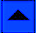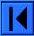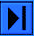Lookup | Welcome | Wiki | Register | Music | Plot 2 | Demos | Index | Browse | More | WebCam
Contribute new seq. or comment | Format | Style Sheet | Transforms | Superseeker | Recent
The OEIS Community | Maintained by The OEIS Foundation Inc.

Last modified October 29 03:28 EDT 2020. Contains 338065 sequences. (Running on oeis4.)